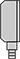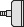﻿ Diaphragm Pressure Gauges | McMaster-Carr
Search Results
 psi in. of H2O oz./in.2
 Air Carbon Dioxide Hydraulic Fluid Natural Gas WaterBottomCenterBack
 2 1/2" 4"
 Steel Stainless Steel
 3 psi 5 psi 10 psi
 Plastic Glass
 10 in. of H2O 15 in. of H2O 30 in. of H2O 60 in. of H2O 100 in. of H2O 160 in. of H2O 200 in. of H2O
 2 in. of H2O 3 in. of H2O 5 in. of H2O 10 in. of H2O 20 in. of H2O 40 in. of H2O
 0.2 in. of H2O 0.5 in. of H2O 1 in. of H2O 2 in. of H2O 4 in. of H2O
 10 oz/sq. in. 15 oz/sq. in. 30 oz/sq. in. 60 oz/sq. in. 100 oz/sq. in. 160 oz/sq. in.
 0.2 oz/sq. in. 0.5 oz/sq. in. 1 oz/sq. in. 2 oz/sq. in. 4 oz/sq. in.
 2 oz/sq. in. 3 oz/sq. in. 5 oz/sq. in. 10 oz/sq. in. 20 oz/sq. in. 40 oz/sq. in.
 0.05 psi 0.1 psi 0.2 psi
 0.5 psi 1 psi 2 psi
Enter e-mail addresses in the fields below
Message